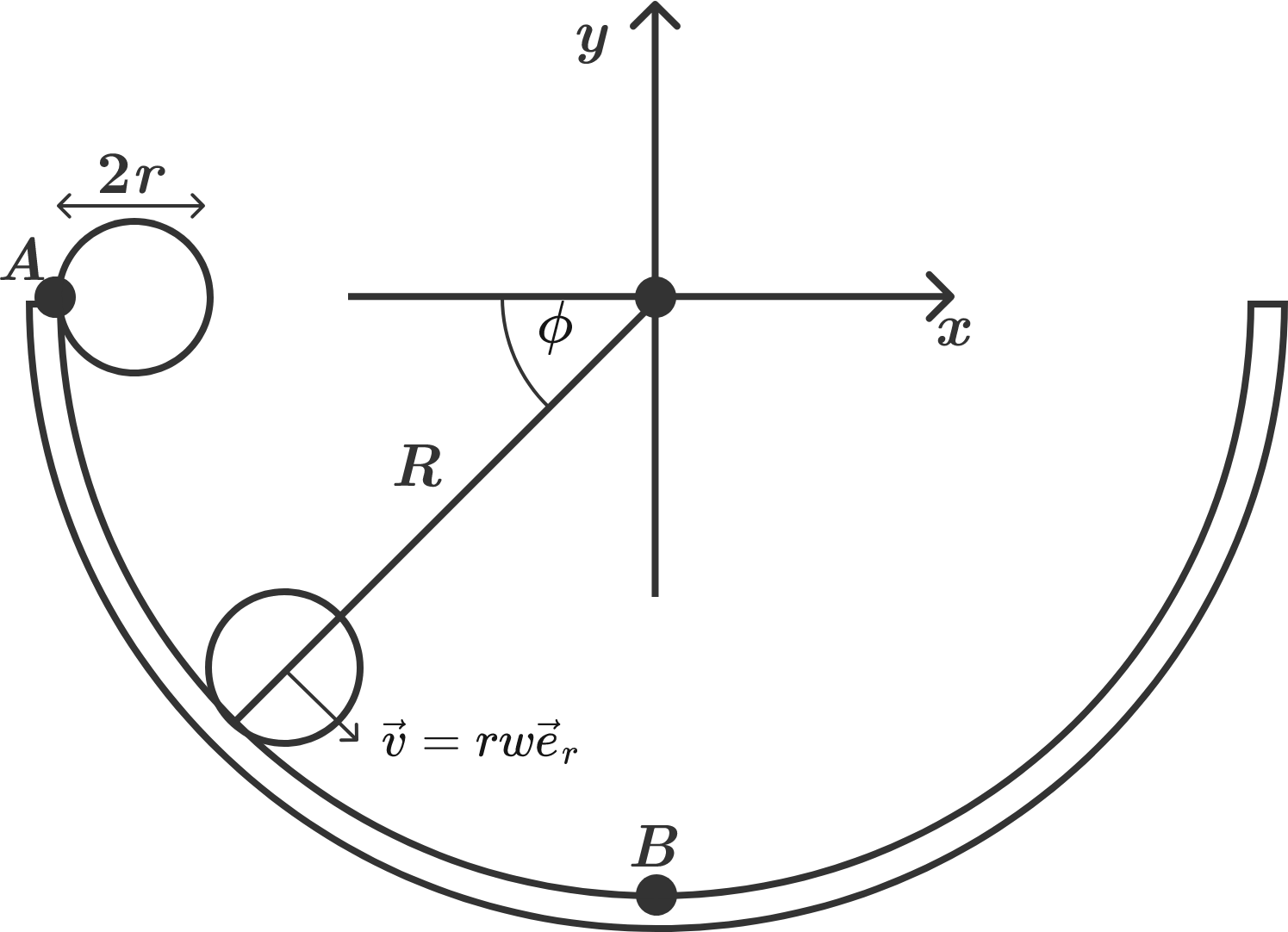# Marble in a bowl

Consider an immovable bowl in the shape of a perfect hemisphere with radius $R = 19 \text{ cm},$ as shown in the diagram below. Now, place a spherical marble with radius $r = 1 \text{ cm}$ on the edge of the bowl $($point $A)$ and let it roll.

How long does the marble take to reach the bottom of the bowl $($point $B)?$Assume that $g = \SI[per-mode=symbol]{10}{\meter\per\second\squared}.$

×